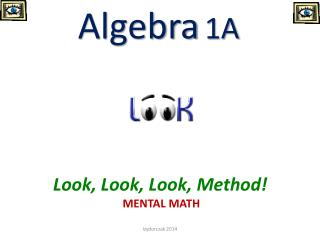# Algebra 1A - PowerPoint PPT PresentationDownload PresentationAlgebra 1A

Algebra 1A
Download Presentation## Algebra 1A

- - - - - - - - - - - - - - - - - - - - - - - - - - - E N D - - - - - - - - - - - - - - - - - - - - - - - - - - -
##### Presentation Transcript

1. Algebra1A Look, Look, Look, Method! MENTAL MATH Izydorczak 2014

2. Mental Math Method for Factoring Second Degree Polynomials Sometimes referred to as reverse FOIL, this method for factoring a second degree polynomial requires you to mentally picture the two binomials that might be factors of the second degree polynomial. For example, suppose you are asked to factor x2 + 5x + 6. The thought process might go something like this: 1)  The sign of the last term (6) is positive.  Therefore the signs of the two binomial factors are the same (since multiplying like signs gives a positive number; if the sign had been negative, we know the binomial factors would have different signs). 2)  The variable parts of the binomial factors are both going to be x, because only x * x (the multiplication of the "firsts") will give me x2. 3)  I know the number parts of the binomials have to be multiply to be 6, since I will be multiplying them together to get the last term of the polynomial.  Factors of 6 are 1 and 6, and 2 and 3. Izydorczak 2014

3. 4)  I know the number parts of the binomials have to add up to 5, because in FOIL, the "OI" parts add up to the x-coefficient.  Which of the factors of 6, that I noted in the step above, add up to 5?  The answer to that is 2 and 3. 5)  So the variable parts of the binomial factors are both x, and the number parts of the binomial factors are 2 and 3.   Therefore my factors are (x + 2)(x + 3). 6)  I always check my work by multiplying the factors I come up with to make sure I come up with the polynomial I started with.  (x + 2)(x +3) = x2 + 5x + 6, so my factors are correct. This method can be used for any polynomial of the form ax2 + bx + c, but it can be tricky to use when a is a value other than 1.  In that case, you have to do extra figuring to determine what combinations of the factors of a and the factors of c will add up to give b.  In cases where a is not 1, it is recommended you use another factoring method, such as factoring by grouping, the box method, the diamond method, or the slide and divide method. Izydorczak 2014

4. x2+ 5x + 6 Look (#1) at sign! 1)  The sign of the last term (6) is positive.  Therefore the signs of the two binomial factors are the same (since multiplying like signs gives a positive number; if the sign had been negative, we know the binomial factors would have different signs). ( + ) ( + ) or ( - ) ( - ) Izydorczak 2014

5. x2+ 5x + 6 2)  The variable parts of the binomial factors are both going to be x, because only x * x (the multiplication of the "firsts") will give me x2. ( + ) ( + ) X X Izydorczak 2014

6. x2+ 5x + 6 3)  I know the number parts of the binomials have to be multiply to be 6, since I will be multiplying them together to get the last term of the polynomial.  Factors of 6 are 1 and 6, and 2 and 3. ( + ) ( + ) Izydorczak 2014

7. x2+ 5x + 6 4)  I know the number parts of the binomials have to add up to 5, because in FOIL, the "OI" parts add up to the x-coefficient.  Which of the factors of 6, that I noted in the step above, add up to 5?  The answer to that is 2 and 3. ( X+ ) (X+ ) 3 2 Izydorczak 2014

8. x2+ 5x + 6 5)  So the variable parts of the binomial factors are both x, and the number parts of the binomial factors are 2 and 3.   Therefore my factors are (x + 2)(x + 3). (x+2) (x+3) Izydorczak 2014

9. x2+ 5x + 6 6)  I always check my work by multiplying the factors I come up with to make sure I come up with the polynomial I started with.  (x + 2)(x +3) = x2 + 5x + 6, so my factors are correct. (x+2) (x+3) CK. x2 + 2x + 3x + 6 x2 +5x + 6 Izydorczak 2014

10. Tryone! Izydorczak 2014

11. x2 - 4x + 4 - - 2 ( ) ( ) X X 2 CK. x2 - 2x - 2x + 4 x2 -4x + 4 Izydorczak 2014